# PROPER Function

Capitalizes the first letter in a text string

## What is the PROPER Function?

The PROPER function is categorized under Excel Text functions. PROPER will capitalize the first letter in a text string and any other letters in text that follow any character other than a letter.

In financial analysis, we often import data from external sources. The PROPER function will help us convert text into the proper case, if required. We can use it to capitalize each word in a given string. Using the function will not affect numbers and punctuations. It will convert all other letters to lowercase.

### Formula

=PROPER(Text)

Text is the required argument. Text needs to be enclosed in quotation marks, a formula that returns text, or a reference to a cell containing the text you want to partially capitalize.

### How to use the PROPER Function in Excel?

The TODAY function was introduced in Excel 2007 and is available in all subsequent Excel versions. To understand the uses of the function, let’s consider a few examples:

#### Example 1

Let’s see how the PROPER function will behave when we give the following formula: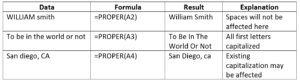We get the results below: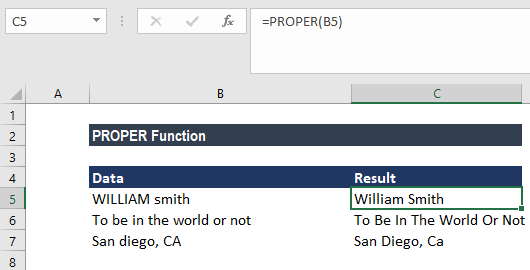#### Example 2

Suppose we wish to clean up names that are not in a proper case, we can use a simple formula based on the PROPER function.

Suppose we are given the data below: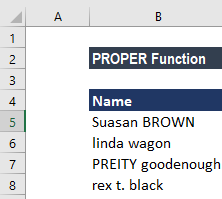The formula to use is: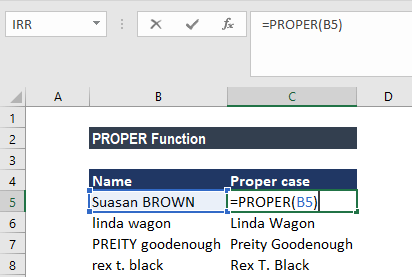We get the results below: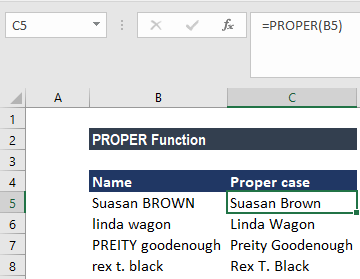The PROPER function will automatically reformat the text so that all words are capitalized. At the same time, it will convert to lowercase all other text.

If we also need to strip out extra spaces in the names, we can wrap PROPER in the TRIM function: =TRIM(PROPER(name). TRIM will remove any leading and trailing spaces, and convert runs of spaces into a single space.

### Things to remember about the PROPER Function

1. The first letter of every word, letters following numbers, or other punctuation are converted to uppercase by the PROPER function. It may be in places that we may not expect. For example, the function converts the “s” in the text string “Bob’s Boat” to uppercase (i.e. “Bob’S Boat”).
2. Numbers and punctuation characters are not affected.

Thanks for reading CFI’s guide to important Excel functions! By taking the time to learn and master these functions, you’ll significantly speed up your financial modeling. To learn more, check out these additional CFI resources:

• Excel Functions for Finance